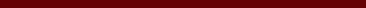### ANALISIS KESTABILAN MODEL EPIDEMI SEIVR PADA PENYAKIT HEPATITIS B

Nurul Subahtul, Toto Nusantara

#### Abstract

In this study, the SEIVR epidemic model was used to analyze the stability of the spread Hepatitis B based on data obtained from the 2016 RI Health Data of East Java Province. In analyzing the model there are several procedures used, namely find the equilibrium point, analyzing the stability of the equilibrium point, find the basic reproduction number (R_0), and the last one doing the simulation based on the data obtained using the Maple17 program. Based on the research that has been done, two equilibrium points are obtained, namely the disease-free equilibrium point and endemic equilibrium point. If the reproduction number R_0<1 then the equilibrium point is stable asymptotically local, and if R_0>1 then the endemic equilibrium point is stable asymptotically local. For the cases of Hepatitis B based on data in the Province of East Java obtained basic reproduction numbers R_0 = 8,648215155x10^(-11) which means Hepatitis B is no longer endemic at any given time.

#### Full Text:

FULL PDF

DOI: http://dx.doi.org/10.17977/um055v1i12020p27-32

### Refbacks

• There are currently no refbacks.

Copyright (c) 2020 Nurul Subahtul, Toto Nusantara